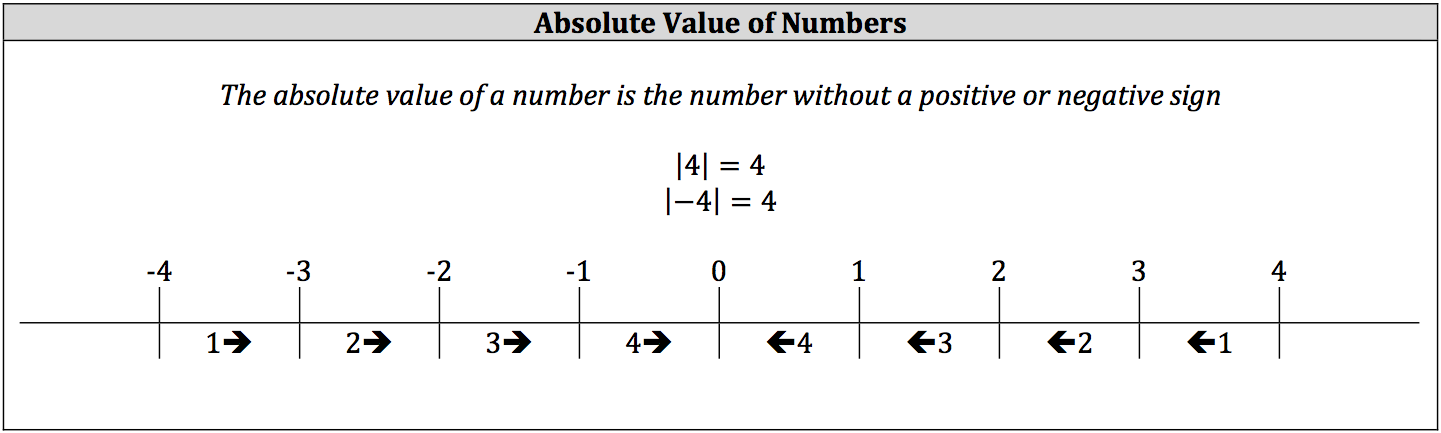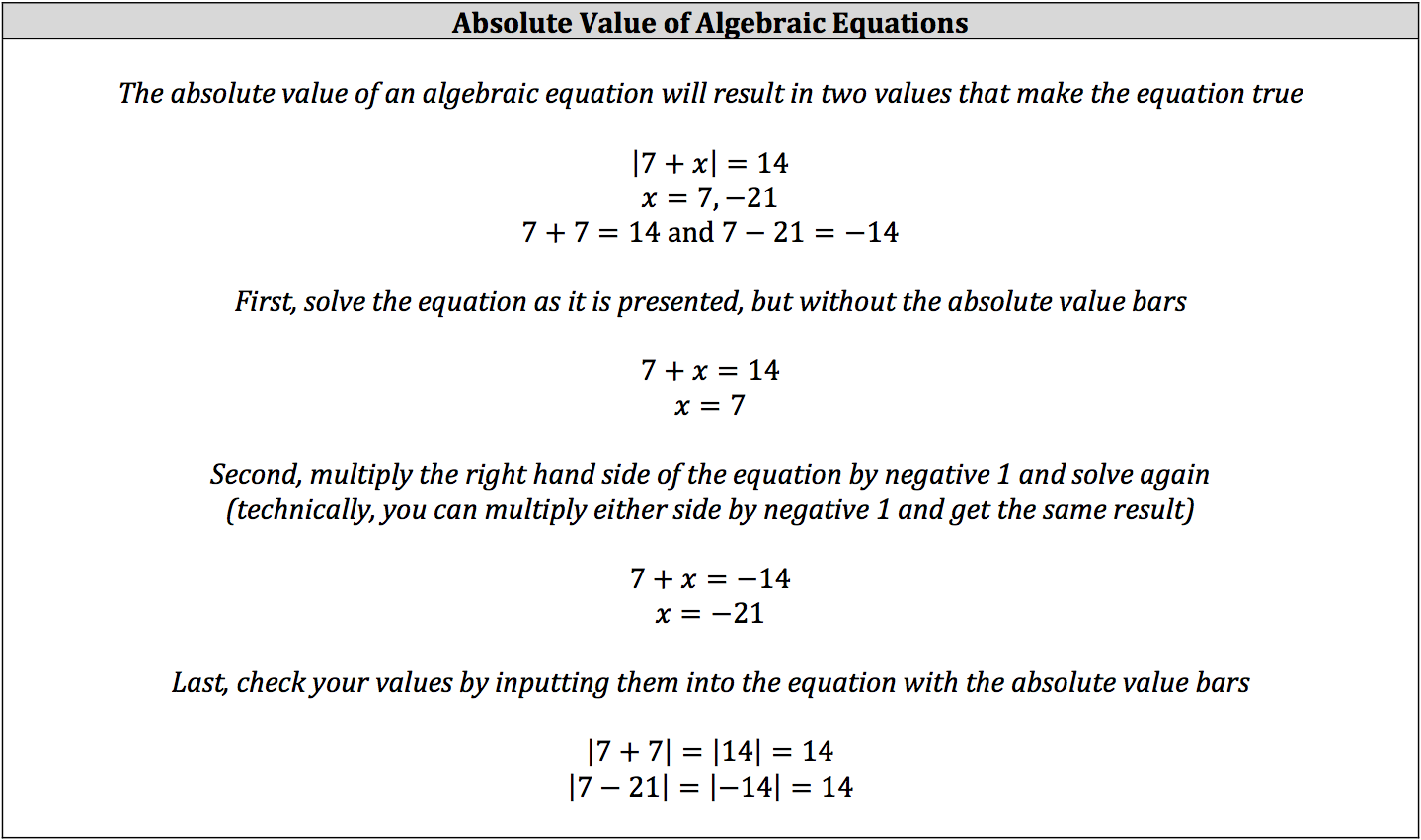Absolute value, represented as a vertical line on either side of the value, refers to a number or variable’s distance from zero on the number line. There are always two numbers that share the same distance from zero: a positive number and its negative counterpart.The absolute value for a variable will usually result in a positive and a negative value as well, but the numbers will not necessarily be counterparts of each other. Instead, the possible values of the variable generate two outcomes where the equation is true.